# Date:8.10.13'm.3mthe origin.(-7.10 Name:Date:8.• Zm10.13'mUse the charges shown:91 = 5 µC92 = -7µC%3D.3m1. Find the value of the electric potential at the origin.(5.10t3 (-2.10yKaiVDart.= r8,99103|4kaz=(6,99:100,2030.7'27=14983= 15000 V0.215000 V.2 Find the electric field at the origin. Draw the electric field vector at the origin on the figure.(6.99.10>2)=1,12.10°(0.2))-1.17.1560.3)"4. 7806.,12X10(8.99.10")(==69927orlt od1.32XW3. What would be the force on q3 = -3 uC if it were placed at the origin?%3D-2.1i +336 53.96 so4. What would bc the potential energy of q3 = -3 µC at the origin?%3D

Question
1 views

Can you help me find the electric field at the origin. I can draw it once I found it.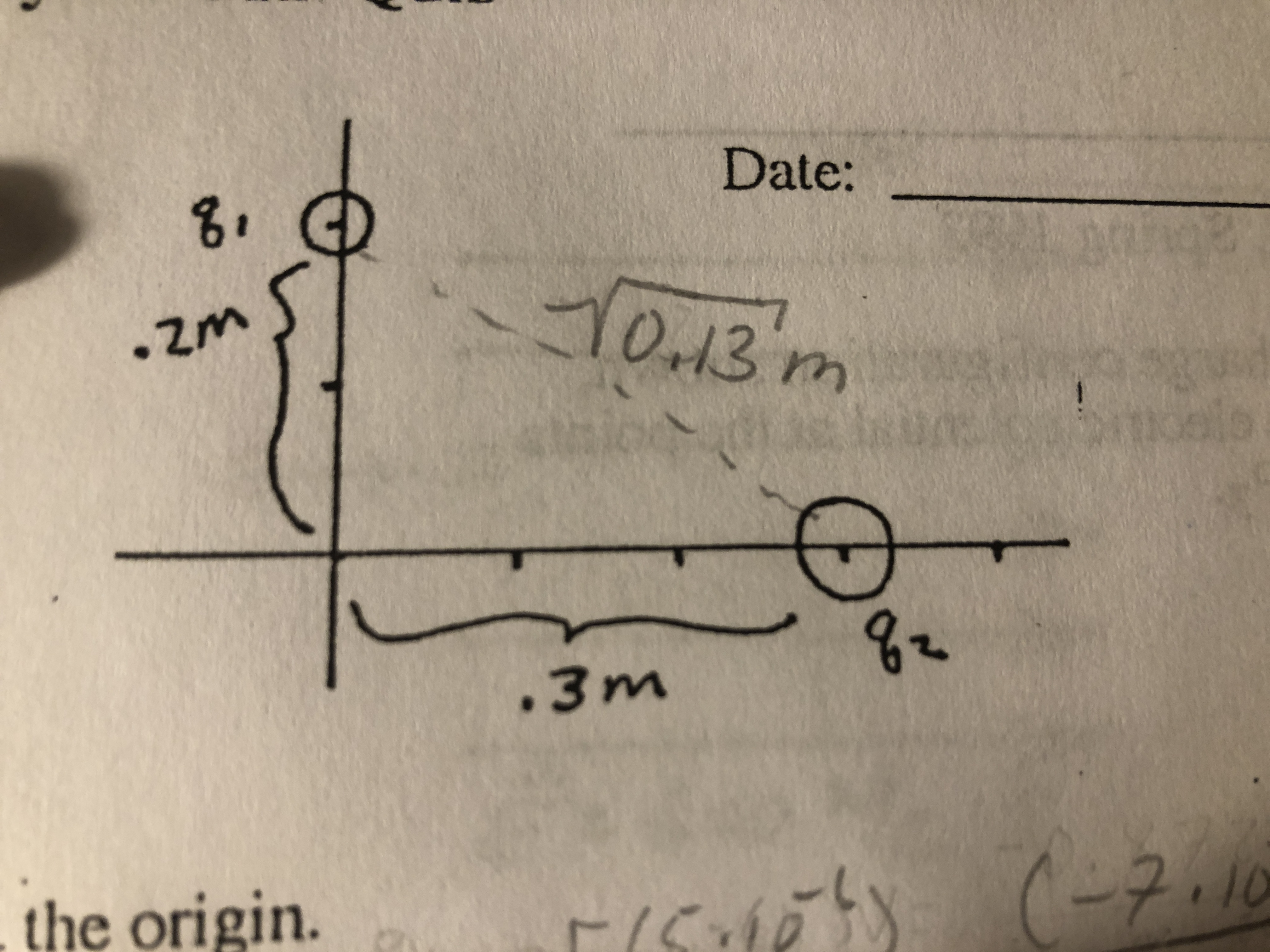help_outlineImage TranscriptioncloseDate: 8. 10.13'm .3m the origin. (-7.10 fullscreen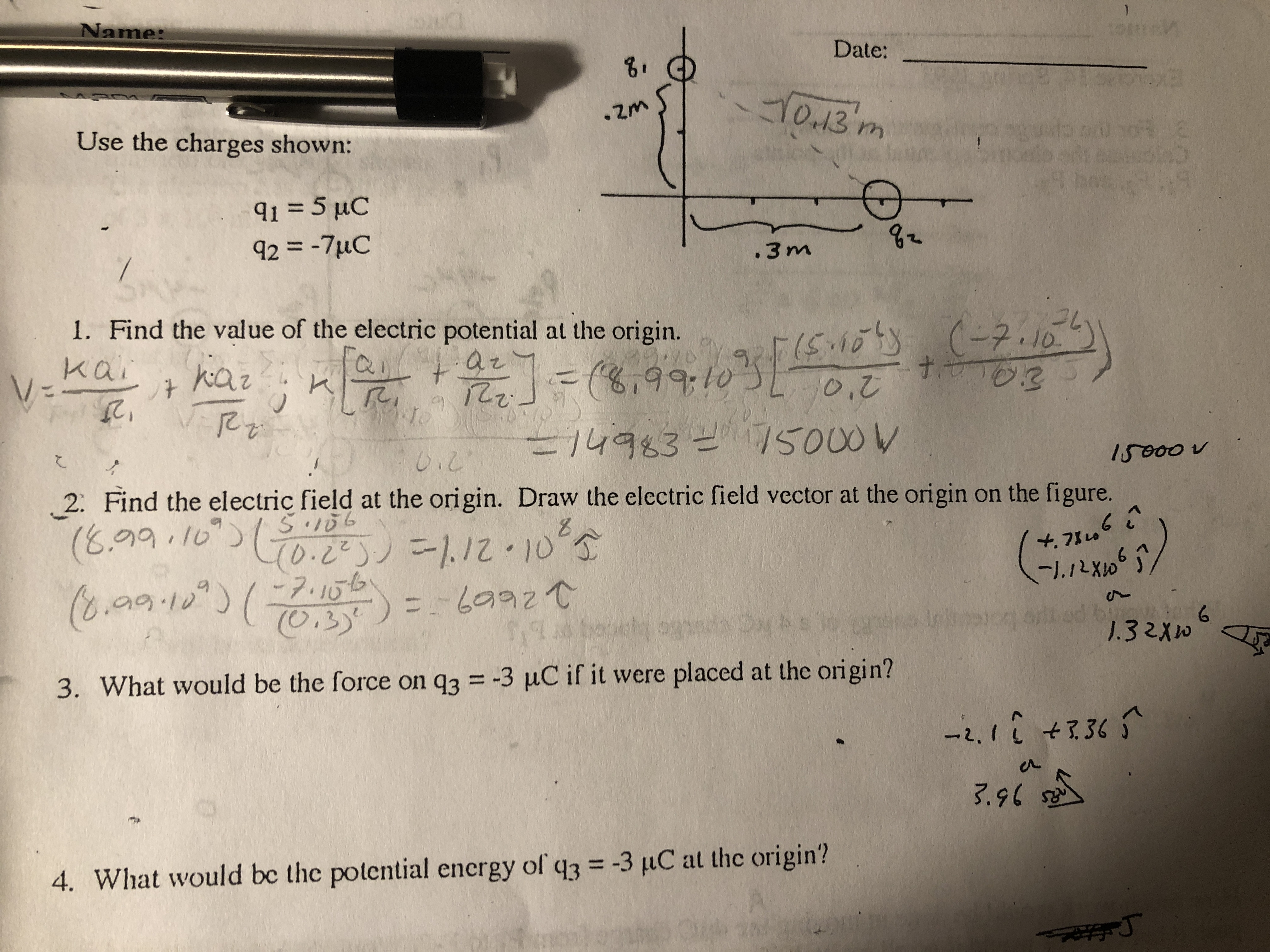help_outlineImage TranscriptioncloseName: Date: 8. • Zm 10.13'm Use the charges shown: 91 = 5 µC 92 = -7µC %3D .3m 1. Find the value of the electric potential at the origin. (5.10t3 (-2.10y Kai VD ar t. = r8,99103|4 kaz =(6,99:10 0,2 03 0.7 '27 =14983= 15000 V 0.2 15000 V .2 Find the electric field at the origin. Draw the electric field vector at the origin on the figure. (6.99.10>2)=1,12.10° (0.2))-1.1 7.156 0.3)" 4. 7806 .,12X10 (8.99.10")( ==69927 orlt od 1.32XW 3. What would be the force on q3 = -3 uC if it were placed at the origin? %3D -2.1i +336 5 3.96 so 4. What would bc the potential energy of q3 = -3 µC at the origin? %3D fullscreen
check_circle

Step 1

(a)

Consider k will be the Columbo’s constant, q is the charge and r is the distance.

The formula for the electric potential is: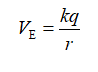Step 2

Since the electric potential is the scalar quantity. Therefore, the electric potential at origin will be: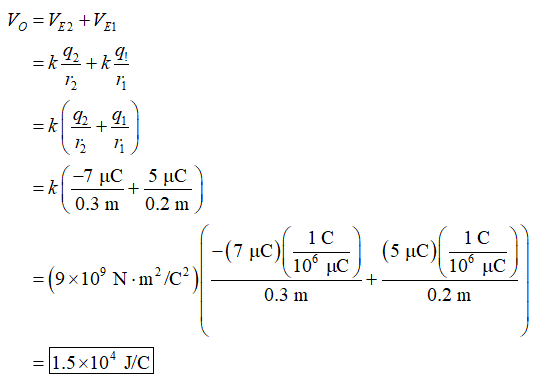Step 3

(b)

The formula for the electric field is:

Since the electric field is a vector quantity. Therefore, the electric field at origin will be: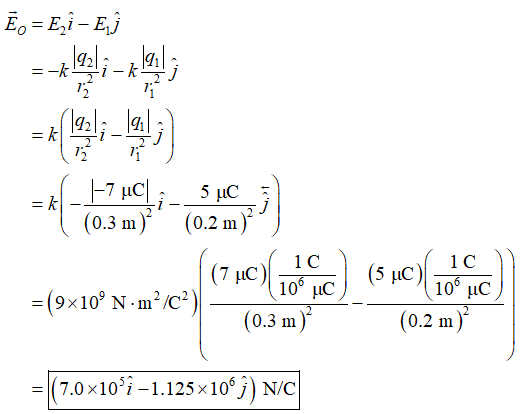...

### Want to see the full answer?

See Solution

#### Want to see this answer and more?

Solutions are written by subject experts who are available 24/7. Questions are typically answered within 1 hour.*

See Solution
*Response times may vary by subject and question.
Tagged in

### Physics